Courses

# Surveying (Part - 2) Civil Engineering (CE) Notes | EduRev

## Civil Engineering (CE) : Surveying (Part - 2) Civil Engineering (CE) Notes | EduRev

The document Surveying (Part - 2) Civil Engineering (CE) Notes | EduRev is a part of the Civil Engineering (CE) Course Civil Engineering SSC JE (Technical).
All you need of Civil Engineering (CE) at this link: Civil Engineering (CE)

Chapter 2 Levelling

LEVELLING
The art of determining relative altitudes of points on the surface of the earth, beneath the surface of the earth is called levelling. This branch of surveying deals with measurement in vertical planes.

LEVEL : The instrument which is used for levelling is known as a level. It consists essentially of the following parts:
(i) A telescope is to provide a line of sight.
(ii) A level tube to make the line of sight horizontal.
(iii) A levelling head to bring the bubble of the tube level at the centre of its run.
(iv) A tripod to support the instrument.

DUMPY LEVEL: It is a simple, compact and stable instrument. The telescope is rigidly fixed to its support and it can neither be rotated about its longitudinal axis and nor it can be removed from its support.Thus it has

(ii) Telescope
(iii) Eye piece
(iv) Diaphragm screws
(v) Focussing-screws
(vii) Longitudinal/Altitude bubble tube
(ix) Cross bubble tube

(i) It is simple in construction with a few movable parts.
(ii) It requires fewer permanent adjustments.
(iii) Adjustment once carried out remains for a longer period.

Levelling Staff : A straight, rectangular wooden rod graduated into meters/feet and further smaller divisions, is called a levelling staff. The bottom of the levelling staff represents the zero- reading. In the most common forms the smallest division is of 5 mm and graduations are in the form of alternate black and white strips.
The purpose of a level (instrument) is to establish a horizontal line of sight. The purpose of the levelling staff is to determine the amount by the which station (i.e. foot of the staff) is above or below the line of sight.

TECHNICAL TERMS USED IN LEVELLING

Level surface : Level surface is a curved surface which is parallel to the mean spheroidal surface of the earth, such that every point on this level surface is equidistant from the centre of the earth. The surface of still water in a lake represents a level surface. Any line lying on the level surface is known as level line.

Horizontal surface : A surface tangential to the level surface at any point is known as a horizontal surface. It is perpendicular to the plumb line at the tangent point. Horizontal line : A line lying on the horizontal surface, is known as horizontal line. It is a straight line tangential to the level line.

Vertical line : A line perpendicular to the level line is called a vertical line. The plumb line at any place, is called the vertical line.
Vertical plane : The plane which contains the vertical line at a place is, called a vertical plane.

Vertical angle : The angle between an inclined line and a horizontal line at a place in vertical plane, is called vertical angle.

DATUM SURFACE :
The imaginary level surface with reference to which vertical distance of  the points (above or below) are measured, is called Datum Surface. In India the datum adopted for G.T.S. Bench marks is the mean sea level at Karanchi.
Mean Sea Level datum: The M.S.L. datum adopted by the survey of India for determining the elevation of different points in India is that of Bombay.

Reduced Level (R.L.): The height or depth of a point above or below the assumed datum is called reduced level (R.L.). It is also known as elevation of the point. Elevations of the point- below the datum surface, are known as negative elevation.
Line of sight: The line passing through the optical centre of the objective, and intersection of cross hairs is known as line of sight.

Bench mark (B.M.): A relatively permanent and fixed reference point of known elevation above the assumed datum, is called a bench mark.

Levelling instrument: Mainly there are two instruments which are commonly used in direct levelling.

(i) A level (Telescope)
(ii) A levelling staff

Special Terms and their Abbreviation Used in Levelling:

1. Instrument station : The point where instrument is set up for observation, is called instrument station.

2. Station : The point where levelling staff is held, is called station. It is the point whose elevation is to be determined.

Height of the instrument (H.I.): The elevation of the line of sight with respect to assumed datum, is known as height of instrument.

Back Sight (B. S.):

The first sight taken on a levelling staff held at point of known elevation is called back sight. It is also known as + ve sight.
Back sight enables the surveyor to obtain the height of the instrument.

Fore sight (F.S.):
The sight taken on a levelling staff held at a point of unknown elevation to ascertain the amount by which the point is above or below the line of sight, is called a foresight.
Fore sight enables the surveyor to obtain he elevation of the point. It is also generally known as minus sight.

Change point:
The point on which both the fore sight and back sight, are taken during the operation of levelling, is called a change point.
It is also called a turning point (T.P.)

Intermediate sight :
The fore sight taken on a levelling staff held at a point between two turning points, to determine the elevation of that point, is known as intermediate sight.
It may be noted that for one setting of a level there will be only a back sight and a fore sight but there can be a number of intermediate sights.

Levelling may be classified as :
1. Simple levelling,
2. Differential levelling.

1. Simple levelling : The operation of levelling for determining the difference in elevation, if not too great between two points visible from a single position of the level, is known as simple levelling.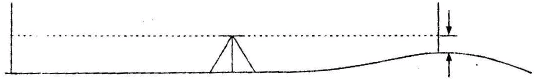2. Differential levelling (fly levelling or continuous levelling) The method of levelling for determining the difference in elevation of two points either too far apart or obstructed by an intervening ground, is known as Differential levelling.
In this method, the level is set up at a number of points and the difference in elevation of successive points is determined as in the case of levelling. This levelling process is also known as Fly compound or continuous levelling.
Suppose that A and B are two points which are far apart and the difference in their elevation, is to be determined by differential levelling.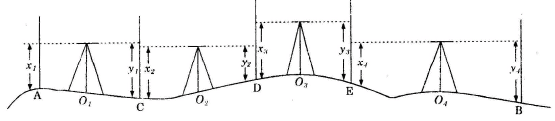Procedure : Following steps are involved:

1. Set up the level at O, ensuring that the line of sight intersects the staff held at A. Level it correctly.
2. With the bubble central, take the back staff reading on the staff held vertically at A.
3. Select a point C equidistant from the instrument position Q, and take the fore sight reading on the staff held vertically at C.
4. Shift the instrument to D, set up and level it correctly.
5. With the bubble central, take the back staff reading on the staff held vertically at C again.
6. Select a point D equidistant from the instrument position Oa and take the fore staff reading on the staff held vertically at D.
7. Repeat the process until the fore staff reading is taken on the staff held on the point B.

Notes :
The following points may be noted :

(i) The points where two readings are taken at the successive points C, D, E, etc. are called change points.
(ii) The level must be set up on firm ground otherwise it may sink during the interval of reading the back and foresight.
(iii) The bubble must always be brought to the centre of its run before staff reading is taken.
(iv). The staff from the change point must not be removed till a back sight is taken. Let x1 x2, x3.........xn be the back sights and y1, y2, y3........ yn be the fore sights on the staff held vertically at A, C, D........etc. Here, Difference of level between A and C = x1 —y1 Difference of level between C and D = x2 —y2 Inference of level between D and E = x3– y3 and so on.
Difference of level of points A and B = S B.S. — S F.S.
If  the difference in level is +ve, the closing point B is higher than starting point A. Whereas if negative,  then point B is lower than the point A.
R.L. of the point B = R.L. of point A ± ( S B.S. — S F.S.)

Booking and Reduction of the Level:

Booking and reduction of the level may be done by following two methods :

1. Rise and fall method.
2. Height of collimation method

Arithmetic check of Rise and fall method : SB.S. — SF.S. = SRise – SFall = Last R.L. – First R.L.
In the rise and fall method of reduction, a complete check on intermediate sights is also provided because these are included for calculating the rises and falls.
Arithmetic Check of Height of Collimation method : B.S. – F.S. = Last R.L. – First R.L.
In this method there is no check on intermediate sights.
Comparison of height of Collimation Method with Rise and Fall method:

Height of collimation method

1. It is more rapid and saves a considerable time and labour.
2. It is well adopted for reduction of levels for construction work such as longitudinal or cross-section levelling operation.
3. There is no check on reduction of R.Ls of intermediate stations.
4. There are only two arithmetic checks i.e. the difference between the sum of back sight and the sum of fore sight must be equal to be the difference in R.L. of last station and first station.
5. Errors if any in intermediate sights are not detected.

Rise and Fall method

1. It is laborious as the staff reading of each station is compared to get a rise or fall
2. It is well adopted for determining the difference in levels of two points where precision is required
3. There is completer check on the reduction of R.Ls of intermediate stations.
4. There are three arithmetical checks is the difference between the sum of the back sights and the sum of fore sights must be equal to the difference between the sum of the rises and the sum of falls as well as it must be equal to the difference in R.Ls of the last station and first station.
5. Errors in intermediate sight are noticed as these are used for finding out rises and fall.

The gradient of a line joining two points, is calculated as given below,

Procedure : Following steps are followed:

1. Calculate the R.L. of each station.
2. Apply usual arithmetic checks to the calculations.
3. Calculate the difference in level of the given two points. i.e., R.L. of the last point – R.L. of the first point.
4. If RL of the last point is more as compared to that of the first point, the gradient is positive i.e. rising gradient. On the other hand, if R.L. of the last point is less than R.L. of the first points, it is a negative gradient i.e. down gradient.
5. Calculate the distance between the end points. It is equal to nd where, n is total number of fore sights and intermediate sight readings on the straight line joining the end points and d is the constant distance between consecutive stations.
6. Divide the distance obtained is step (5) by the difference in level obtained in step (3), to obtain the desired gradient.

Readings recorded in a level book may be classified into two categories:
(1) Basic reading: The reading which are observed by a level on a levelling staff  held vertically at the starting B.M. and other intermediate stations, are known as basic readings, such as back sight, intermediate sights, fore sights and the R. L. of the get bench marks.
(2) Derived readings : The reading which are derived with the help of the basic readings, are known as the derived readings such as rises, falls, the height of collimation and R.L.s of unknown points.

Spirit Levelling classification of:
(1) Differential levelling
(2) Check levelling
(3) Reciprocal levelling
(4) Profile levelling
(5) Cross-sectional levelling
(6) Precision levelling

Differential levelling: The operation of levelling to determine the elevation, reduced - level of points at some distance apart to establish Bench Marks in the area, is called differential levelling or fly levelling or simple fly levelling.
Profile levelling : The operation of levelling carried out to determine the elevations of the points at known distances apart, and also other salient features, along a given straight line is called profile levelling or longitude levelling.
Reciprocal levelling: The operation of levelling in which difference in elevations between the points is determined by two sets of reciprocal observations, is called reciprocal levelling. 

• Reciprocal levelling is employed when it is not possible to set up the level between two points due to an intervening obstruction such as large water bodies.
• It is used to obtain accuracy and a eliminate the following :
(ii) combined effect of earth’s curvature and the refraction the atmosphere, and
(iii) variations in the average rejection.

Procedure

(I) When level is near to A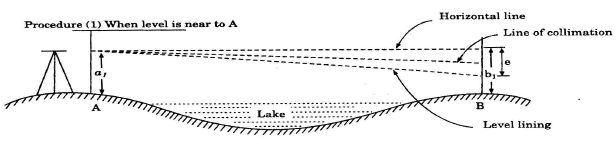(II) When level is near to B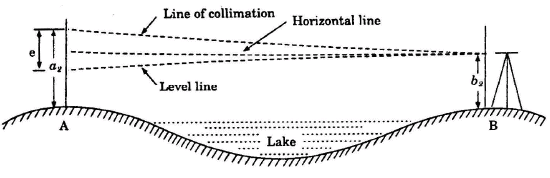Let h = true of levels between A and B e = combined error due to refraction, curvature and imperfect adjustment of the line collimation.
First Position of the level:

The correct reading on staff B = b1– e. The correct reading on staff A = a1

Assuming A to be higher than B, the true difference of level h = b– e – a1 or h = (b2 – a2) + e

Adding equations (i) and (ii), we have 2h = (b1–a1) + (b2 – a2

True difference of level between A and B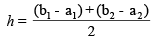The true difference of level between A and  B is equal to the mean of the two apparent different of level.
The combined error can be obtained by equating the equations (i) and (ii),

(b1 – a1) – e = (b2–a2) + e

2e = (b1–a1) – (b2 – a2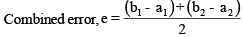i.e., combined error is equal to the half of the difference of the apparent differences of levels.
NOTE:
(i) In reciprocal levelling, the collimation error and the error due to curvature are completely eliminatied.
(ii) The elimination of the error due to refraction depends upon the change in the climatic conditions during the transfer of the instrument. (iii) A set of observations at different times of the day, may be made to obtain the accurate difference of level by taking the mean of all the differences. Difference of levels by taking the mean of all the differences.
Curvature correction: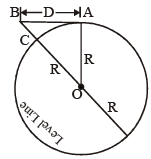Let AB = d, the horizontal distance between A and OC = OA = R , the radius of earth BC = Cc, the correction for the curvature from the right angled DBAO, we get

BO2 = OA+ AB2
or (OC +  BC)2 = OA+ AB2

or (R + CC)2 = OA+ AB2
or R2 + 2RCC + CC2 = R2 + d2
or CC (2R + CC) = d2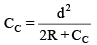But CC is negligible as compared to the diameter of the earth, therefore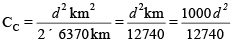correction for curvature is, negative and is always substracted from the staff reading.

\ Correction for refraction,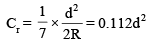Hence correction for curvature is, negative and is always added to the staff reading.

Correction due to curvature and refraction.
Correction due to curvature =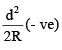Correct due to refraction =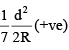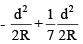Hence, combined correction due to curvature and refraction =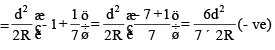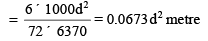Combined correction due to curvature and refraction = 0.0673 d2 m where d is in km.

Note:
(i) For ordinary lengths of sight, the error due to curvature and refraction is very small and is generally ignored.
(ii) The error due to curvature and refraction can be eliminated by equalizing back sight and fore sight distance. i.e., by balancing the sights.
(iii) The error due to curvature and refraction can also be eliminated by the method of reciprocal levelling.

Offer running on EduRev: Apply code STAYHOME200 to get INR 200 off on our premium plan EduRev Infinity!

## Civil Engineering SSC JE (Technical)

109 docs|50 tests

,

,

,

,

,

,

,

,

,

,

,

,

,

,

,

,

,

,

,

,

,

;